Search IntMath
Close

# Tanzalin Method for easier Integration by Parts

By Murray Bourne, 12 Apr 2010

Here's a rather neat way to perform certain integrations, where we would normally use Integration by Parts method.

Tanzalin Method can be easier to follow (and could be used to check your work if you have to do Integration by Parts in an examination).

Tanzalin Method is commonly used in Indonesia. I can't find a reference to it anywhere else (in the English literature) and I couldn't find any information on Tanzalin, presumably a mathematician.

## Example 1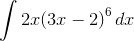#### Integration by Parts Method

First, let's see normal Integration by Parts for comparison.

We identify u, v, du and dv as follows:

 u = 2x dv = (3x − 2)6dx du = 2dx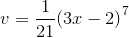Integration by Parts then gives us: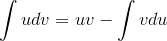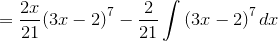Now, we find the unknown integral: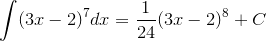Putting it together, we have: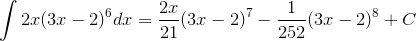We can then factor and simplify this to give: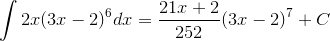The Tanzalin Method is somewhat less messy.

#### Example 1, now using Tanzalin Method

In the Tanzalin Method, we set up a table as follows. In the first column are successive derivatives of the simplest polynomial term of our integral. (We need to choose this term for the derivatives column because it will disappear after a few steps.)

In the second column are the integrals of the second term of the integral.

We just multiply the 2 terms with green background in the table (the original 2x term and the first integral term). We don't change the sign of this term.

We then multiply the 2 terms with yellow background (the first derivative and the second integral term). We assign a negative sign to the product, as shown.

The answer for the integral is just the sum of the 2 terms in the final column.

The question again, for reference: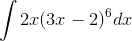Derivatives Integrals Sign Same-color Products
2x (3x − 2)6
2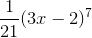+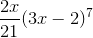0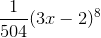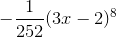Summing the 4th column:(We add the constant of integration, C only at the end, not in the table.)

We can then factor and simplify this to give:## Example 2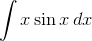We'll go straight to the Tanzalin Method.

Derivatives Integrals Sign Same-color Products
x sin x
1 −cos x + x cos x
0 −sin x sin x

We multiplied (x) by (−cos x) and we didn't change the sign.

We then multiplied (1) by (−sin x) and changed the sign.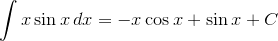## Example 3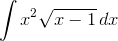Using the Tanzalin Method requires 4 rows in the table this time, since there is one more derivative to find in this case.

We need to alternate the signs (3rd column), so our 4th row will have a positive sign.

Derivatives Integrals Sign Same-color Products
x2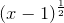2x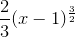+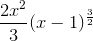2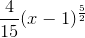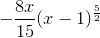0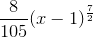+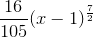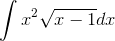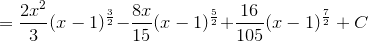## Example 4 - a Problem Arises

This is the same question as Example 3 in the Integration by Parts section in IntMath.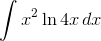We need to choose ln 4x (natural logarithm of 4x) for the first column this time, following the Integration by Parts priority recommendations of:

1. log of x,
2. x raised to a power
3. e raised to power x

[Note: If we choose the other way round, we would have to find integrals of ln 4x, which is not pretty (and certainly no easier than doing it all using Integration by Parts. See Example 6 on this page: Integration by Parts).

Derivatives Integrals Sign Same-color Products
ln 4x x2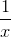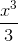+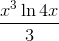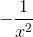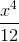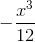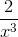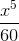+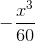When do we stop? The derivatives column will continue to grow, as will the integrals column. The Tanzalin Method requires one of the columns to "disappear" (have value 0) so we have somewhere to stop.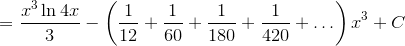That expression in brackets must equal 1/9 (since this is the answer we got using Integration by Parts), but as you can see, it is not a Geometric Progression and would take some figuring out.

## Conclusion

While Tanzalin Method only handles integrals involving (at least one) polynomial expressions, it is worth considering as a simpler way of writing Integration by Parts questions.

### Comment Preview

HTML: You can use simple tags like <b>, <a href="...">, etc.

To enter math, you can can either:

1. Use simple calculator-like input in the following format (surround your math in backticks, or qq on tablet or phone):
a^2 = sqrt(b^2 + c^2)
(See more on ASCIIMath syntax); or
2. Use simple LaTeX in the following format. Surround your math with $$ and $$.
$$\int g dx = \sqrt{\frac{a}{b}}$$
(This is standard simple LaTeX.)

NOTE: You can mix both types of math entry in your comment.